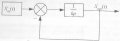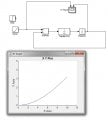# Automatic control theory: structural diagram in MATLAB-simulink.

Thread Starter

#### Cyrenaica —

Joined Jun 7, 2015
17
hi !

never experienced such a programm . now i have to model an extreme simple system , structural circuit of what is in the picture below , but i am not sure if everything is correctthe initial conditions are:
1) Xin(t) = 1(t) <<< is signal a ramp then or a pulse ?
2) feedback is negative <<< ok
3) lets assume k EQU 12, s EQU p <<< ok

i need to :
1) build circuit properly in matlab <<< c pic below
2) build graph Xout(t) = f[Xin(t)] <<< which jack of "XY Graph" is for y ?hope for fast response

#### Russmax

Joined Sep 3, 2015
82
Cyrenaica,
The circuit certainly looks to be in order. On the XY Graph box, Xin goes to X, and Xout goes to Y. But you have not been asked to plot the transfer function H(s) = Xout(s)/Xin(s)

I don't understand item 1) in your initial conditions, Xin(t) = 1(t). What does that mean? Does it mean Xin(t) = 1 or Xin(t) = t ? I'm guessing that it means Xin(t) = 1.

If my assumption is correct, they want you to plot the response of the system to a step function. In other words, hook of the step function and plot Xout(t).

Regards

Similar threads